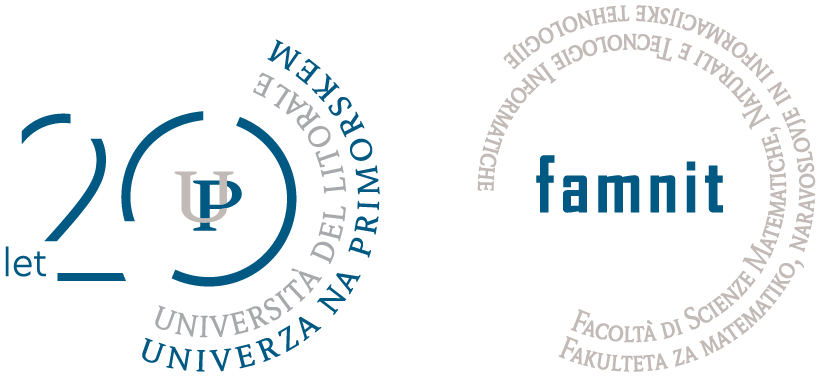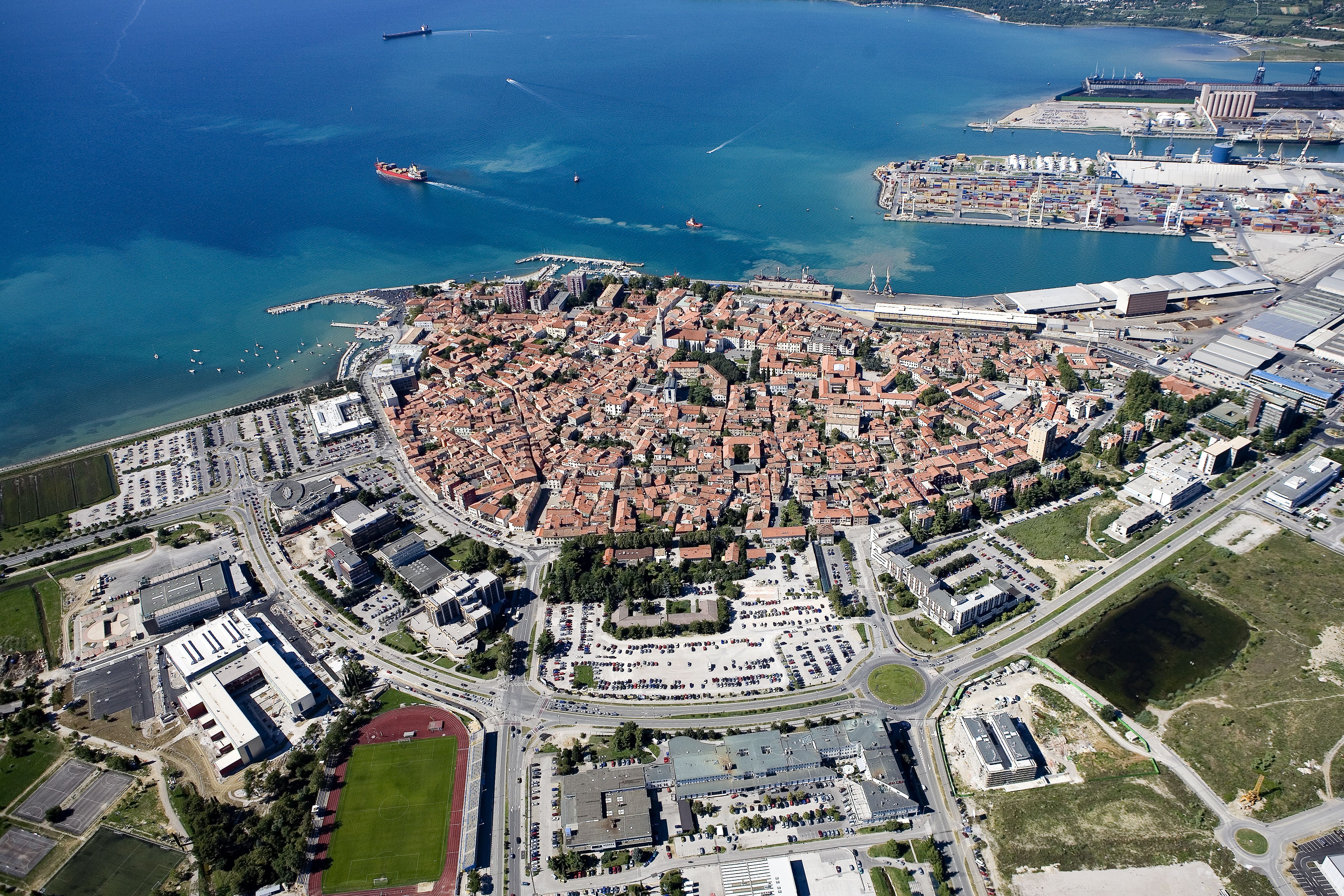Univerza na Primorskem Fakulteta za matematiko, naravoslovje in informacijske tehnologijeSI | EN# Raziskovalni matematični seminar - Arhiv

 2023 2022 2021 2020 2019 2018 2017 2016 2015 2014 2013 2012 2011 2010
 1 2 3 4 5 6 7 8 9 10 11 12
Datum in ura / Date and time: 20.12.12
(14:00-15:00)
Predavalnica / Location: Famnit-Semin (Seminar room)
Predavatelj / Lecturer: Prof. Andreas Brandstädt (University of Rostock, Germany)
Naslov / Title: On the complexity of some packing and covering problems in certain graph classes
Vsebina / Abstract:

Packing and covering problems in graphs and hypergraphs and their relationships belong to the most fundamental topics in combinatorics and graph algorithms and have a wide spectrum of applications in computer science, operations research and many other fields. Vertex Cover is a typical example of a covering problem, and Maximum Independent Set is a typical example of a packing problem, and the complexity of these two problems was investigated in thousands of papers.

Recently, there has been an increasing interest in problems combining packing and covering properties. For a hypergraph H = (V, E), Exact Cover is a classical problem asking for the existence of a subcollection E′ ⊆ E such that every vertex of V is contained in exactly one hyperedge from E′. It is well known that this problem is NP- complete even when the hyperedges contain only three vertices called Exact Cover by 3-Sets.

Among the problems combining packing and covering properties, there are the follo- wing variants of domination problems: Let G = (V, E) be a finite undirected and simple graph, and let N(G) denote the closed neighborhood hypergraph of G. A vertex set U ⊆ V is an efficient dominating set in G if the closed neighborhoods of vertices in U form an exact cover in N (G). An edge set M ⊆ E is an efficient edge dominating set in G if M is an efficient dominating set in the line graph L(G). Note that not every graph has an efficient dominating set (an efficient edge dominating set, respectively). The problem Efficient Domination (Efficient Edge Domination, respective- ly) asks for the existence of such a vertex set (edge set, respectively). It is well known that both problems are NP-complete, even for bipartite graphs. We give some new ca- ses where the problems are efficiently solvable by using structural properties of graph classes and using closure properties under the square operation, and we also generalize the problems to hypergraphs.

Datum in ura / Date and time: 11.12.12
(10:00-11:00)
Predavalnica / Location: FAMNIT-SEMIN
Predavatelj / Lecturer: prof. Pablo Spiga (University of Milano-Bicocca)
Naslov / Title: Is the theory of finite primitive groups finished?
Vsebina / Abstract:
` From time to time you hear people saying that the theory of finite primitive groups is dead (after theO'Nan-Scott theorem and the Classification of the Finite Simple Groups). In this talk, we present a result on cycle lengths that could have been discovered by Schur, or Wielandt or Manning. However, they didn't because such powerful results are not the end of the theory, but the beginning.`

Datum in ura / Date and time: 3.12.12
(10:00)
Predavalnica / Location: FAMNIT-MP
Predavatelj / Lecturer: Dragan Marušič
Naslov / Title: Primitive groups of degree twice a prime number (part II)
Vsebina / Abstract:

We will give a short overview and discuss possible future directions with regards to the classification of strongly regular bicirculants, in relation to the problem of obtaining a CFSG-free proof of nonexistence of simply primitive groups of degree \$2p\$.